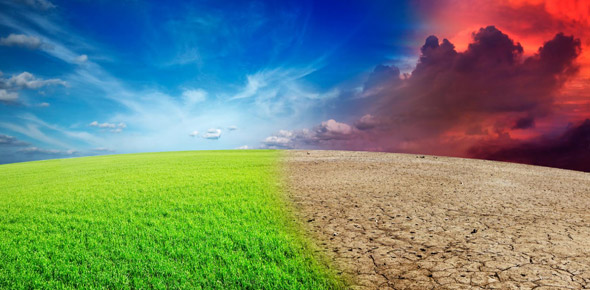# States Of Matter And Changes Of State Trivia Quiz

10 Questions | Total Attempts: 613SettingsCreate your own QuizStates of Matter and Changes of State

• 1.
A _______ change occurs when a substance changes phase.
• A.

Physical

• B.

Chemical

• 2.
A change of phase from vapor to liquid is called ______.
• A.

Evaporation

• B.

Condensation

• C.

Distillation

• D.

Vaporization

• 3.
When a substance dissolves, it undergoes a ______.
• A.

Chemical change

• B.

Physical change

• C.

Molecular change

• 4.
As a change of state is occurring, the temperature of the system does not change.
• A.

True

• B.

False

• 5.
The change of phase from a solid to a gas is called _____.
• A.

Evaporating

• B.

Melting

• C.

Sublimation

• D.

Vaporization

• 6.
Which of the following statements is true?
• A.

Unsaturated solutions will not dissolve in more solute.

• B.

For most substances, the solid state is more dense than the liquid state.

• C.

Density is not temperature dependent.

• D.

A nonpolar solute will dissolve in a polar solvent such as water.

• 7.
Which of the following is the best name for a compound made from calcium and bromine (CaBr2)?
• A.

Calcium bromide

• B.

Calcium dibromide

• C.

Monocalcium dibromide

• D.

Calcium bromine II

• 8.
Which of the following is a properly written formula for a compound between copper and bromine?
• A.

CuBR

• B.

CUBR

• C.

CuBr

• D.

CUBr

• 9.
Which of the following is the best name for a compound made from nitrogen and oxygen (N2O3)?
• A.

Nitrogen oxide

• B.

Nitrogen II oxide

• C.

Nitrogen trioxide

• D.

Dinitrogen trioxide

• 10.
Which part of an atom is actively exchanged or shared in a chemical bond?
• A.

Proton

• B.

Electron

• C.

Neutron

• D.

Depends on the type of atom

Related TopicsBack to top
×

Wait!
Here's an interesting quiz for you.